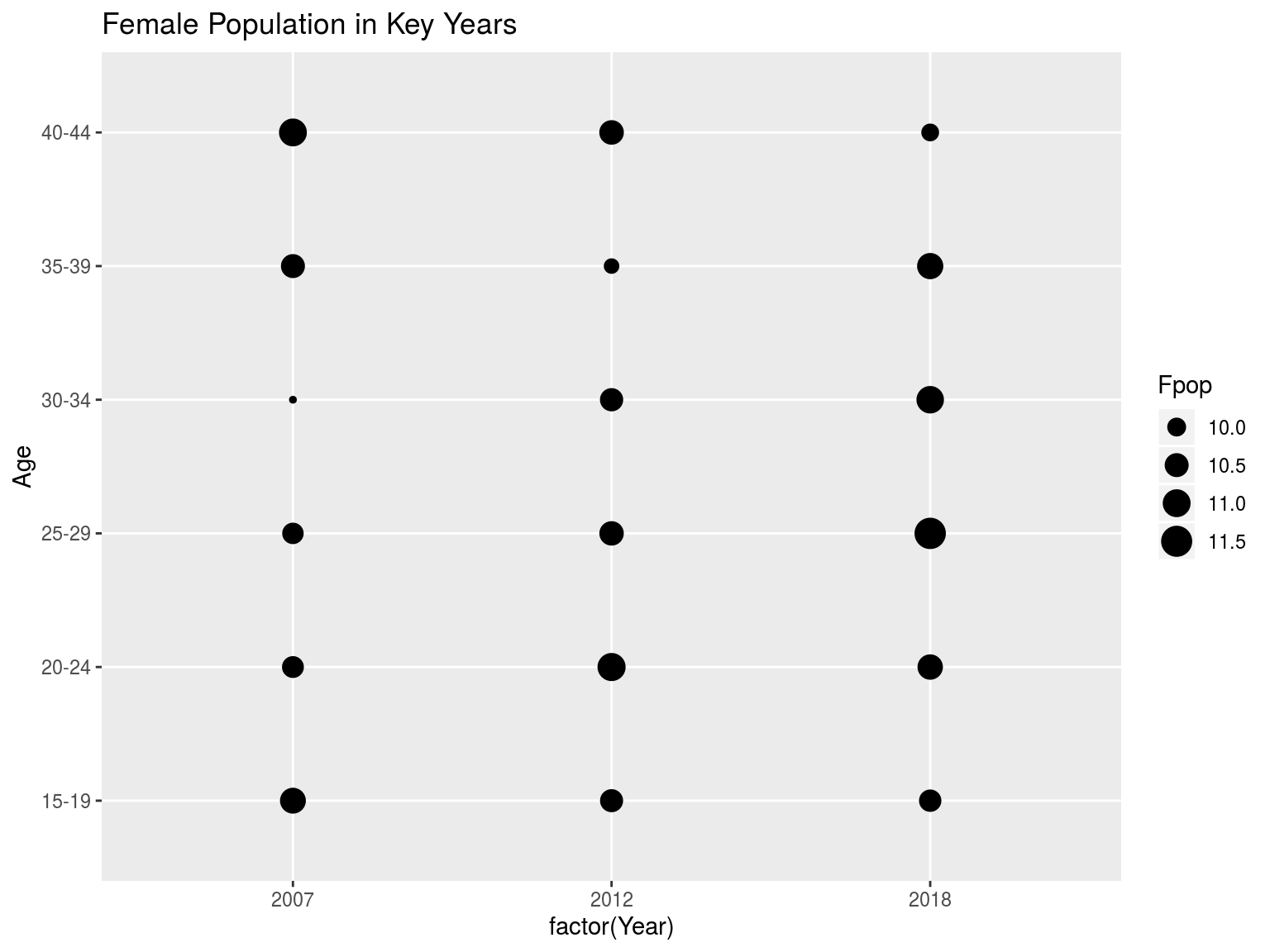2/28/2020

# Libraries and Data

library(tidyverse)
## ── Attaching packages ───────────────────────── tidyverse 1.3.0 ──
## ✓ ggplot2 3.2.1     ✓ purrr   0.3.3
## ✓ tibble  2.1.3     ✓ dplyr   0.8.4
## ✓ tidyr   1.0.2     ✓ stringr 1.4.0
## ✓ readr   1.3.1     ✓ forcats 0.4.0
## ── Conflicts ──────────────────────────── tidyverse_conflicts() ──
## x dplyr::lag()    masks stats::lag()
load("/cloud/project/Nat0718.Rdata")

# Problem

Show graphically the number of births in the US from 2007 forward.

BirthsByYear = Nat0718 %>%
mutate(Year = Year %% 1000,
Births = Births/1000000) %>%
group_by(Year) %>%
summarize(Births = sum(Births)) %>%
ungroup()

BirthsByYear %>% ggplot(aes(x=Year,y=Births)) +
geom_point() +
ggtitle("Millions of US Births by Year")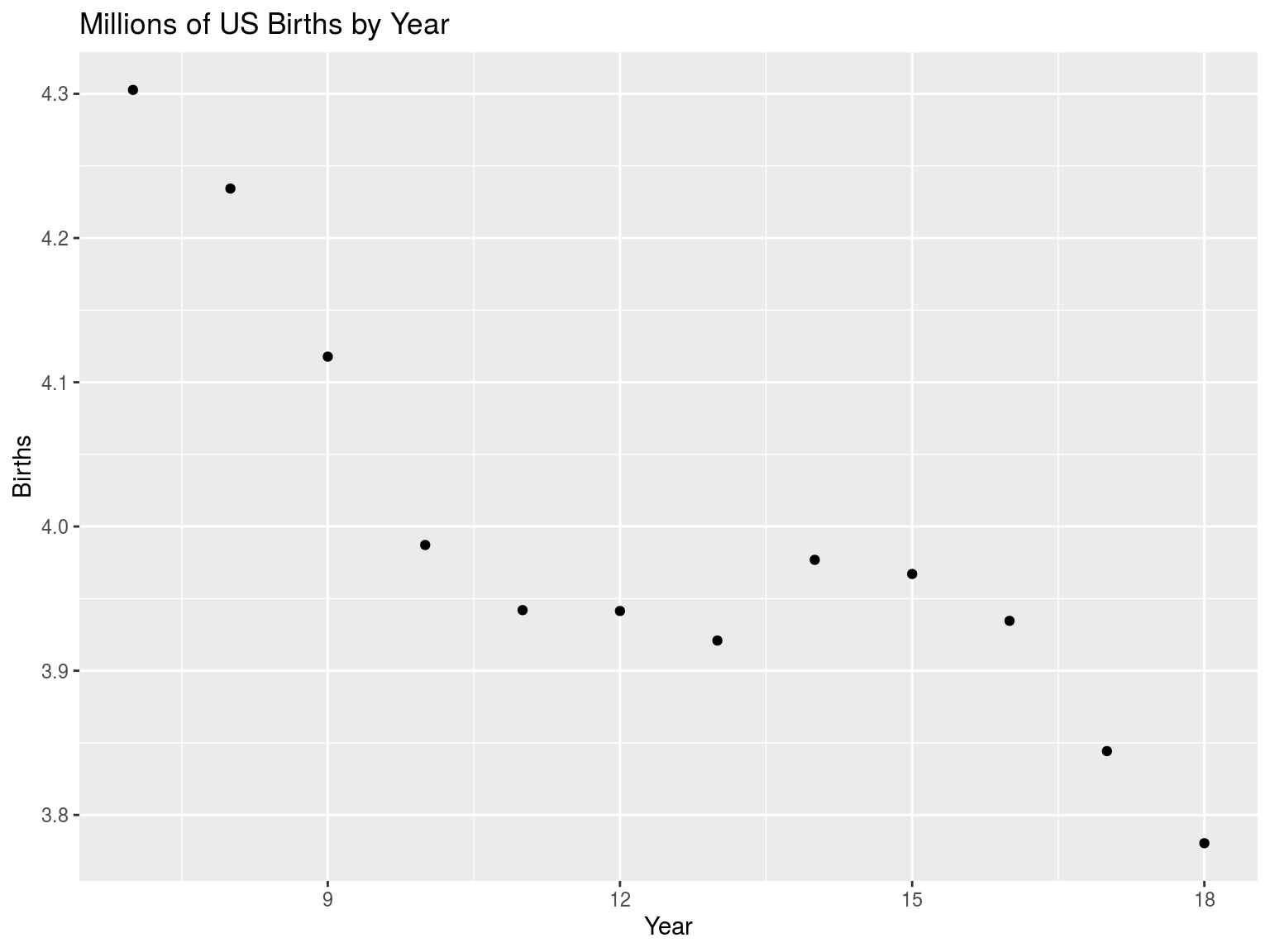# Problem

The observed pattern could be caused by a shift of the numbers of women in the relevant age groups rather than a change in childbearing behavior.

To pursue that line of thought, show graphically what has happened to the total fertility rate over the same period. Show both the absolute value and the ratio of the current TFR to its maximum value.

TFRByYear = Nat0718 %>%
mutate(Year = Year %% 1000) %>%
group_by(Year,Age) %>%
summarize(Births = sum(Births),
Fpop = sum(Fpop)) %>%
mutate(Rate = Births/Fpop) %>%
ungroup() %>%
group_by(Year) %>%
summarize(TFR = sum(Rate) * 5) %>%
mutate(Ratio = TFR/max(TFR)) %>%
ungroup()

TFRByYear %>% ggplot(aes(x=Year,y=TFR)) +
geom_point() +
ggtitle("US TFR by Year")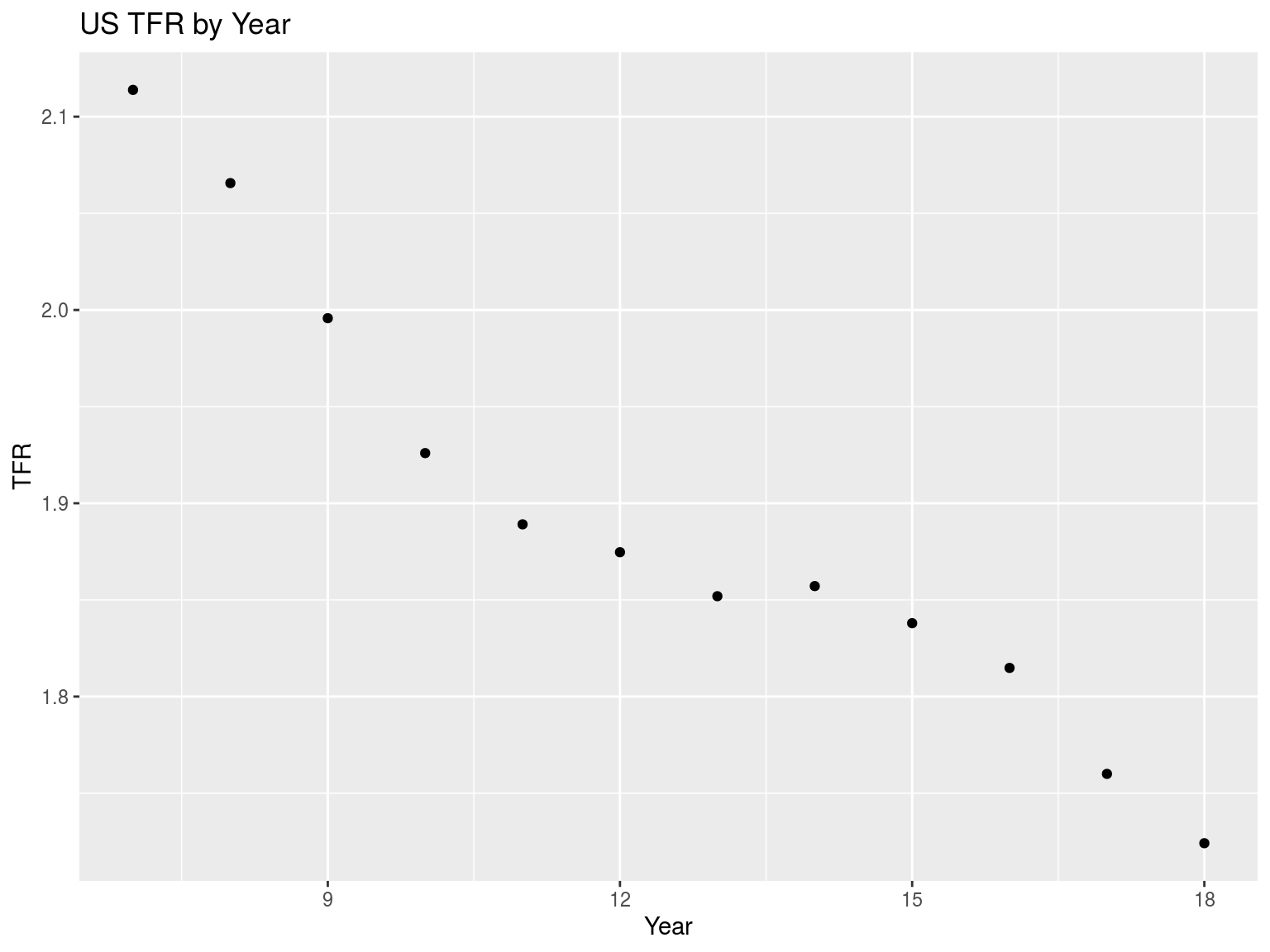TFRByYear %>% ggplot(aes(x=Year,y=Ratio)) +
geom_point() +
ggtitle("US TFR by Year (Ratio)")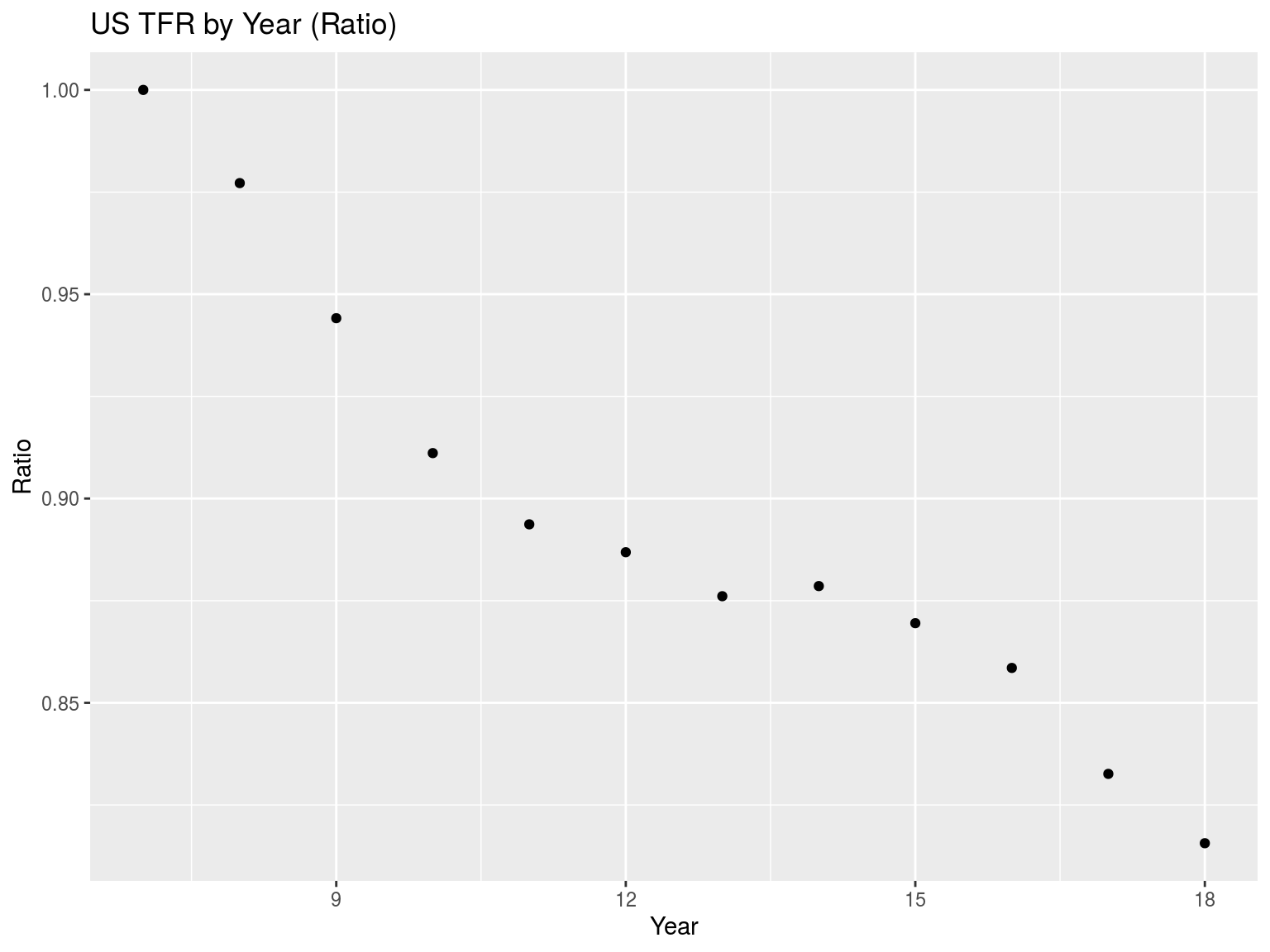# Problem

The future values of the TFR might be larger than the current value if there is a delay in childbearing rather than an absolute change in the number of births per woman.

To pursue that line of thought, examine the trends in birthrates for the five-year age groups separately. Look for a reduction in the younger age groups and a possible increase in the older age groups. Use the ratio-to-maximum value for an age group over time to do this.

BirthRateByAgeYear = Nat0718 %>%
mutate(Year = Year %% 1000) %>%
group_by(Year,Age) %>%
summarize(Births = sum(Births),
Fpop = sum(Fpop)) %>%
mutate(Rate = Births/Fpop) %>%
ungroup() %>%
group_by(Age) %>%
mutate(Ratio = Rate/max(Rate)) %>%
ungroup()

BirthRateByAgeYear %>%
ggplot(aes(x=Year,y=Ratio)) +
geom_point() +
facet_wrap(~Age) +
ggtitle("Birthrates by Age and Year (Ratio)")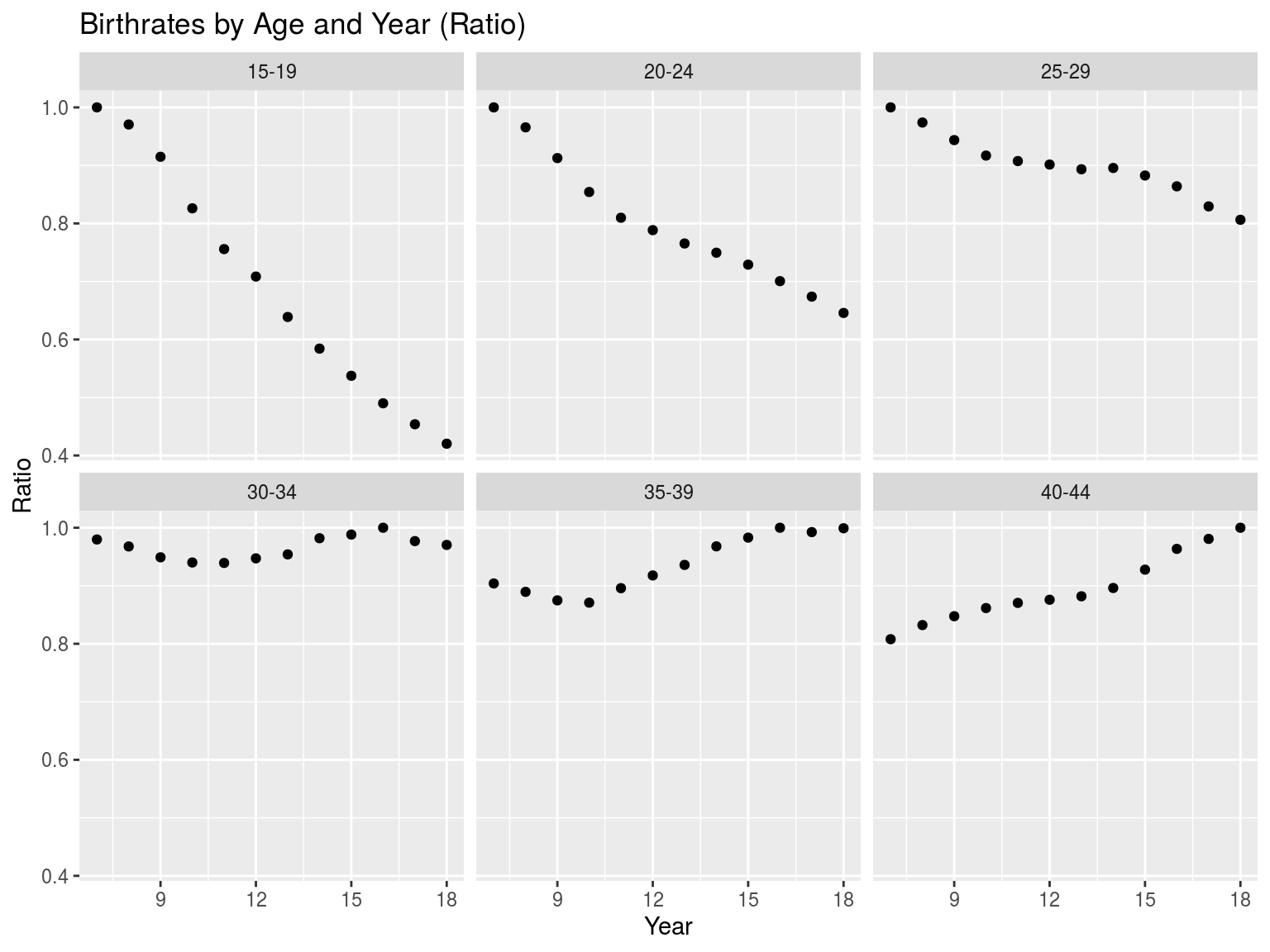# Problem

For any of these age groups, births are the product of the number of women and the corresponding birthrate. Show graphically what hahappened to the sizes of these age groups during this time period. Use actual values in millions.

FemalePopByAgeYear = Nat0718 %>%
group_by(Age,Year) %>%
summarize(Fpop = sum(Fpop)/1000000) %>%
ungroup()

FemalePopByAgeYear %>%
ggplot(aes(x=Year,y=Fpop)) +
geom_point() +
facet_wrap(~Age) +
ggtitle("Female Population (millions)")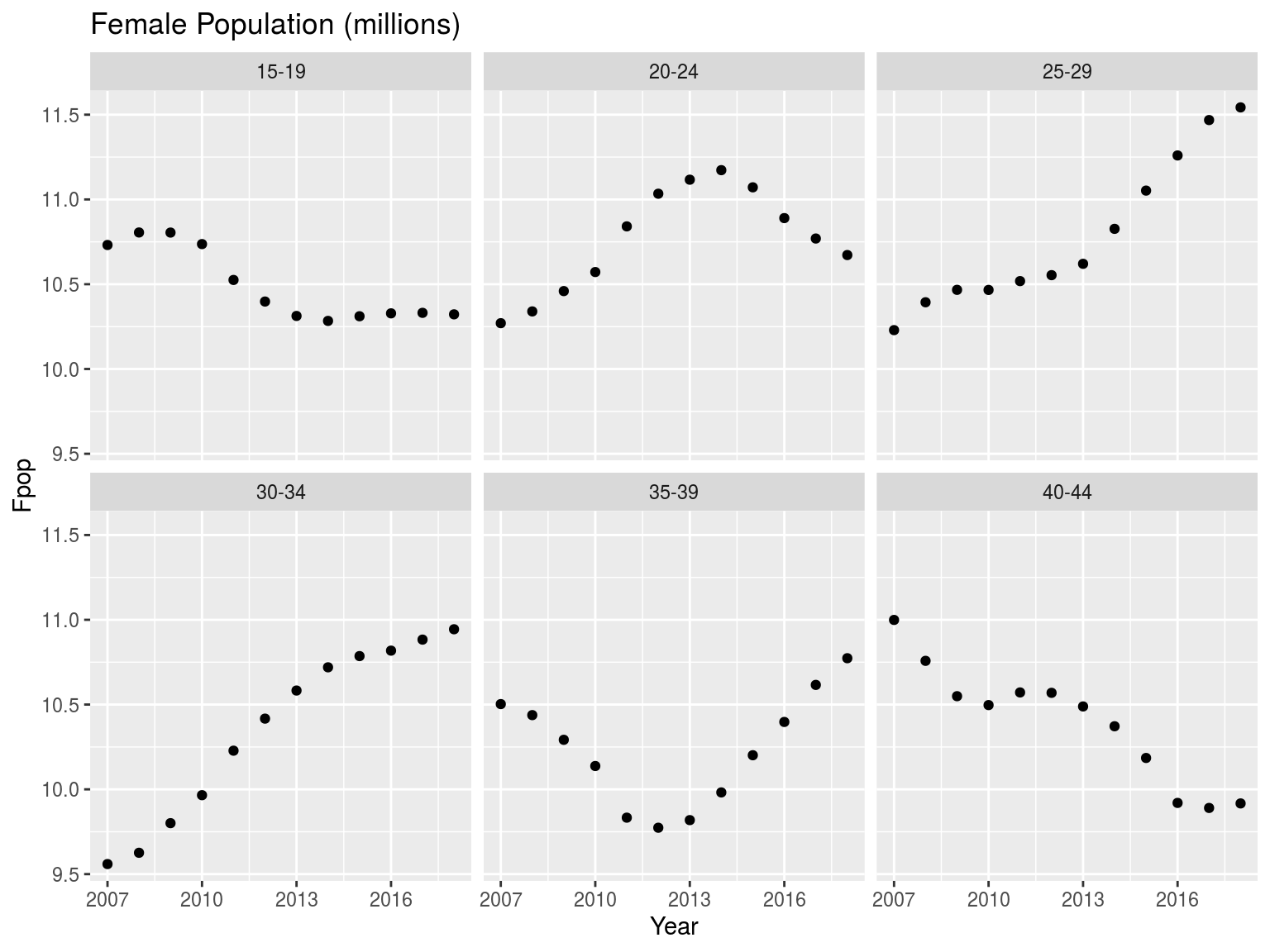# Problem

Show in one graph how the age structure of the relevant female population has differed for a few key years. Use 2007, 2012 and 2018.

FemalePopByAgeYear %>%
ggtitle("Female Population in Key Years")